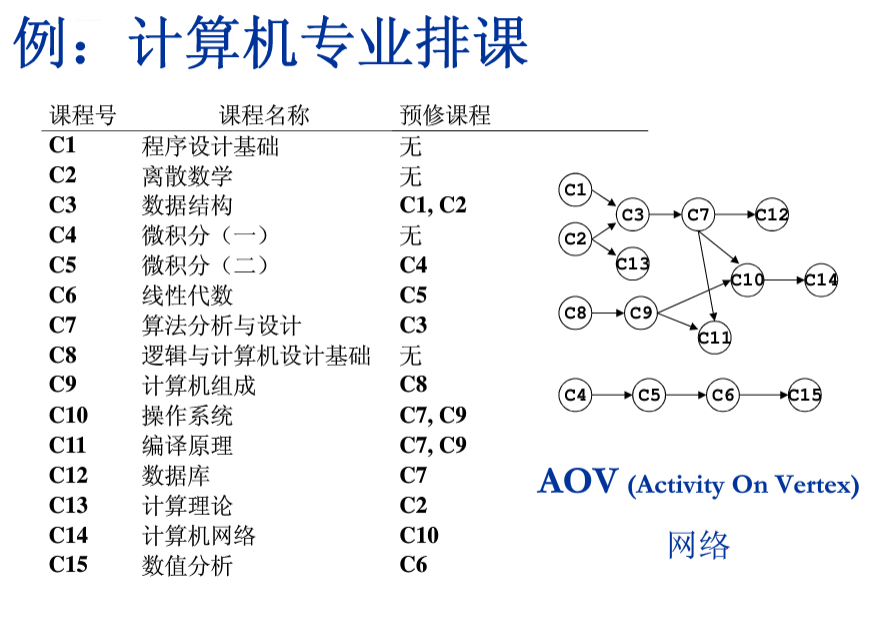# 拓扑排序

###### 拓扑排序理解###### Description

Aiden陷入了一个奇怪的梦境：他被困在一个小房子中，墙上有很多按钮，还有一个屏幕，上面显示了一些信息。屏幕上说，要将所有按钮都按下才能出去，而又给出了一些信息，说明了某个按钮只能在另一个按钮按下之后才能按下，而没有被提及的按钮则可以在任何时候按下。可是Aiden发现屏幕上所给信息似乎有矛盾，请你来帮忙判断。

3 3
1 2
2 3
3 2

T_T
2

###### Data Size & Hint

#include<iostream>
#include<vector>
#include<queue>
using namespace std;
const int N = 10010;
const int INF = 0x3f3f3f3f;
int n,m;
int indegree[N];
int map[N][N];
vector<int>ans;	//存储答案
void Init()  //初始化
{
for(int i = 1; i <= n; i++){
for(int j = 1; j <= n; j++){
map[i][j] = -1;
}
}
}

void topsort()
{
queue<int>q;  //存储入度为0的点
for(int i = 1; i <= n; i++){
if(!indegree[i]) q.push(i);  //如果入度为0，则加入队列
}
while(!q.empty()){
int temp = q.front();
q.pop();
ans.push_back(temp);     //将可按下的按钮存放到ans中
for(int i = 1; i <= n; i++){
if(map[temp][i] != -1){
indegree[i]--;
if(indegree[i] == 0){
q.push(i);
}
}
}
}

}

int main()
{
cin>>n>>m;
int x,y;  //只有按下x按钮后才能按y
Init();
for(int i = 1; i <= m; i++){
cin>>x>>y;
map[x][y] = 1;
indegree[y]++;//入度+1
}
topsort();
if(ans.size() == n){  //全部按下
cout<<"o(∩_∩)o"<<endl;
} else{
cout<<"T_T"<<endl;
cout<<n-ans.size()<<endl;
}
return 0;
}©️2019 CSDN 皮肤主题: 数字20 设计师: CSDN官方博客